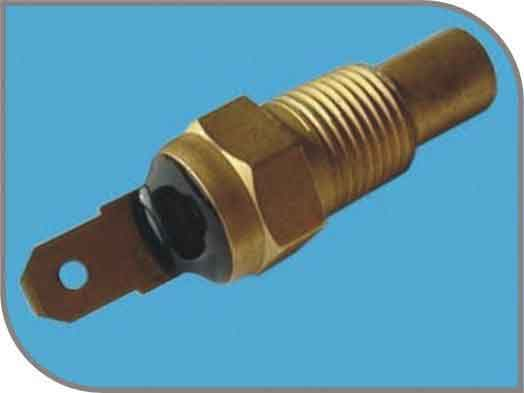﻿ What is the significance of the linear NTC temperature sensor temperature interchange accuracy parameter?_

China sensor manufacturers

# What is the significance of the linear NTC temperature sensor temperature interchange accuracy parameter?

What is the significance of the linear NTC temperature sensor temperature interchange accuracy parameter?
Interchange accuracy refers to the ideally determined straight line for the same determination under the same working conditions (same working current, same temperature field). The voltage V(T) of each sensor—the maximum deviation of the temperature T curve from the line. This deviation is usually expressed by the temperature-voltage conversion coefficient S of the sensor. Due to the linearization of the output of the sensor and the same temperature-voltage conversion factor. That is, the whole process is interchanged in the temperature range, so the interchange accuracy indicates the degree of dispersion of the reference voltage value. That is, the discrete value of the reference voltage value is converted into a temperature value to describe the degree of interchange between the entire batch of sensors. Generally divided into three levels: the exchanging deviation of the I level is not more than 0.3 ° C; the J level is not more than 0.5 ° C; the K level is not more than 1.0 ° C.﻿
﻿